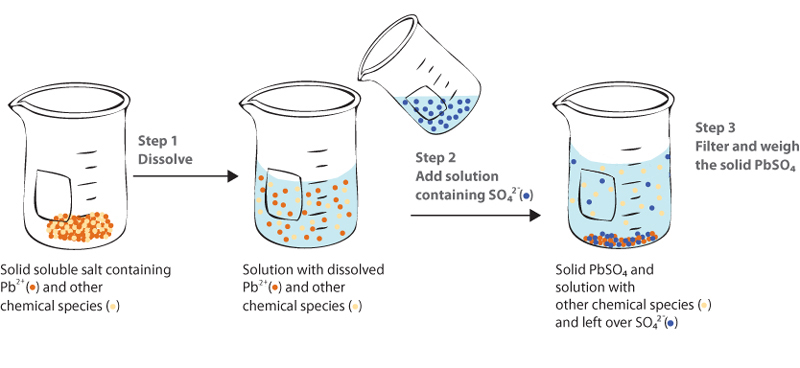# Gravimetric Analysis

-Introduction

-Precipitating the Pb2+ ion

-Amount of Pb2+ in the sample

-Application Question

-Evaluation Questions

Experiment 1 Online Tutorial >> Gravimetric Analysis >> Introduction

 Next >

# Experiment 1 - Gravimetric Analysis

## Introduction

In this experiment, your goal is to determine the amount of lead present in a water sample and, if lead is present, to determine whether the level of contamination is potentially dangerous. Preliminary preparation of the sample has resulted in a solid of unknown composition. This solid contains lead (Pb2+) in the form of a water soluble salt and gravimetric analysis will be used to determine the amount of lead present.

The experimental strategy is shown schematically below.We start with a solid that contains some lead(II) ions (Pb2+, shown as brown dots) along with other chemical species (shown as yellow dots). For the purposes of this analysis, the precise identity of the other chemical species is not important. Our goal is to somehow separate the lead(II) from these other species and then, once isolated, measure the amount of lead(II). We do this through the following 3 steps:

Step 1

Action: We dissolve the solid. We use an acidic solution to prevent formation of solid Pb(OH)2. (Since the concentration of OH- in an acidic solution is small, we suppress formation of the Pb(OH)2.)
Result: All species that were in the solid are now in the solution, including the Pb2+ and the other chemical species.

Step 2

Action: We add a solution containing SO42-. We must add enough SO42- to precipitate all of Pb2+, we add excess SO42-. Any excess SO42- remains dissolved in the solution and does not interfere with the remainder of the experiment.
Result: A solid that is essentially pure PbSO4 is formed. The other chemical species remain dissolved in solution.
Note: This step takes advantage of the fact that SO42- forms an insoluble salt with only a few ions, of which Pb2+ is the most common. (Ksp at 25°C for PbSO4 is 1.6 x 10-8, making it quite insoluble). We therefore assume that the only chemical species that will form a solid when we add SO42- to the solution is Pb2+, and so the solid we have after step 2 is pure PbSO4.

Step 3

Action: Filter the solution and weigh the solid PbSO4.
Result: From the mass of the PbSO4, we can determine the amount of Pb2+ in our final PbSO4 solid. Since all of the Pb2+ in the original solid ended up in our final PbSO4, we have determined the amount of Pb2+ in the initial sample.

The following pages contain tutors to assist you in the calculations required for the above steps. Please click next to continue to the tutors.

 Next >